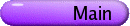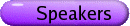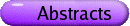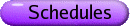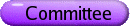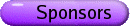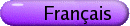Ergodic Theory
Org: Christopher Bose (Victoria) and Andres del Junco (Toronto)
[PDF]

WAEL BAHSOUN, University of Victoria
Deterministic representation for position dependent random maps
[PDF]

We give a deterministic skew-type representation for position dependent random maps and describe the structure of the set of its invariant densities. We prove one-to-one correspondence between absolutely continuous invariant measures (acims) for the position dependent random map and the acims for its skew product representation.

ADAM FIELDSTEEL, Wesleyan University, Middletown, CT, USA
Relative pressure and the variational principle
[PDF]

We define notions of pressure associated with topological and measure-theoretic factors of compact dynamical systems and prove a variational principle: The topological quantity is the supremum of the set of the corresponding measure-theoretic quantities. A central device is the use of average (or [`(d)]-) separation of orbits in the definition of pressure, in place of the classical notion of separation. This change permits arguments that are, we hope, simplifications of the classical arguments in the proofs of variational principles, and which can readily be extended to obtain analogous results for actions of amenable semigroups.

This is joint work with Russell Coe, CCSU.

NIKOS FRANTZIKINAKIS, Penn State University
Sets of multiple recurrence
[PDF]

An integer subset set S is a set of k-recurrence if for every measure preserving system and measurable set A with positive measure, the sets A,T-nA,...,T-knA intersect on a set of positive measure for infinitely many n Î S. Furstenberg constructed an example of a set of 1-recurrence but not 2-recurrence. For every positive integer k we will give explicit examples of sets of k-recurrence but not (k+1)-recurrence (joint work with E. Lesigne and M. Wierdl). We will also discuss the question of whether the set of shifted primes is a set of k-recurrence (joint work with B. Host and B. Kra).

EMILY GAMBER, UNC-Chapel Hill, CB #3250, Chapel Hill, NC 27599-3250
Topological Properties of D-dimensional Cellular Automata
[PDF]

A cellular automaton is a tool used to model complex systems, making discrete simulations of an intricate process. Cellular automata were first investigated from a purely mathematical point of view in 1969 with Hedlund's formative paper. This work was motivated by then-current problems in symbolic dynamics, possibly those of a cryptographic nature. When Wolfram turned his attention to cellular automata via computer simulation in the early 1980s, the subject gained momentum. Wolfram categorized one-dimensional cellular automata based on features of their asymptotic behavior which could be seen on a computer screen. Gilman's work in 1987 and 1988 was the first attempt to mathematically formalize these characterizations of Wolfram's; he utilized the notions of equicontinuity and expansiveness, as well as measure theoretic analogs of each. While measure is intrinsic to Gilman's partition, Kurka has a purely topological classification centered on equicontinuity, expansiveness, and sensitivity. We extend the one-dimensional topological classification of Kurka for cellular automata on the full shift space, to higher dimensional subshift spaces, providing examples to highlight the differences between one- and two-dimensional cellular automata, as some results do not extend directly from one dimension.

THIERRY GIORDANO, University of Ottawa, Ottawa
Connes and Woods Approximate Transitivity and Dimension Spaces
[PDF]

In 1985, Connes and Woods defined the notion of an approximately transitive (AT) action of a group on a Lebesgue space in their study of the flow of weights of particular von Neumann factors, the Araki-Woods factors or equivalently of the associated flow of ergodic, non-singular transformations. Four years later, they studied the Poisson boundary of group-invariant, time-dependent Markov random walks defined on a locally compact group G and pointed out that it is an AT and amenable G-space. G. A. Elliott and myself proved that any AT and amenable G-space is such a Poisson boundary. With D. Handelman, I have introduced the notion of dimension G-spaces to study AT and amenable G-actions. In this talk, I will review the notion of approximate transitivity and of dimension G-spaces and present some specific constructions of AT and non-AT transformations.

PAWEL GORA, Concordia University, Montreal
Properties of invariant densities of random maps
[PDF]

We show a few results about the existence of absolutely continuous invariant measure (acim) for random maps both with constant probabilities and with probabilities dependent on position. Then, we discuss some properties of the acim's. In particular we show an example of a random map satisfying Pelikan's condition with the support of acim consisting of infinite number of disjoint intervals and with the density of acim not separated from 0. This is in contrast with the properties of acim's for individual piecewise expanding maps.

GUANGYUE HAN, University of British Columbia
Analyticity of Hidden Markov Chains
[PDF]

We prove that under a mild positivity assumption the entropy rate of a hidden Markov chain varies analytically as a function of the underlying Markov chain parameters. We give examples to show how this can fail in some cases. And we study two natural special classes of hidden Markov chains in more detail: binary hidden Markov chains with an unambiguous symbol and binary Markov chains corrupted by binary symmetric noise. Finally, we show that under the positivity assumption the hidden Markov chain itself varies analytically, in a strong sense, as a function of the underlying Markov chain parameters.

SHAFIQUL ISLAM, University of Lethbridge
Approximation of absolutely continious invariant measures for random dynamical systems
[PDF]

A random map is a discrete-time dynamical system in which one of a number of transformations is randomly selected and applied at each iteration of the process. The asymptotic properties of a random map are described by its invariant densities. I will talk about Ulam's method for approximation of absolutely continious invariant measures for Markov switching position dependent random maps. Piecewise linear approximation of absolutely continious invariant measures for random maps will also be discussed.

DAVE MCCLENDON, University of Maryland
Orbit discontinuities of Borel semiflows on Polish spaces
[PDF]

Let X be a standard Polish space. Given an action Tt of [0,¥) by (presumably non-invertible) Borel maps on X, we say that two distinct points x and y are "instantaneously discontinuously identified" (IDI) if Tt(x) = Tt(y) for all t > 0. Such phenomena is an obstacle to representing the action as a shift map on a space of continuous paths. We define the concept of "orbit discontinuity", a generalization of IDI, and discuss results regarding the structure and prevalence of such behavior. In particular, the set of points which are IDI has measure zero with respect to any measure preserved by the semiflow and is invariant under Borel time changes.

This material is part of my Ph.D. research conducted under the direction of Dan Rudolph.

RANDALL MCCUTCHEON, University of Memphis, Memphis, TN 38152, USA
Central sets and multiple recurrence for non-amenable group actions
[PDF]

Measurable multiple recurrence results for non-nilpotent groups have up to now been limited to an ergodic Roth theorem of Bergelson, McCutcheon and Zhang, which states that for any measure preserving actions {Tg}g Î G and {Sg}g Î G of a countable amenable group G on a probability space (X,B,m) that commute in the sense Tg Sh = Sh Tg for all g,h Î G, and any A Î B with m(A) > 0, limn [ 1/(|Fn|)]åg Î fn m(AÇTg-1 AÇ(Tg Sg)-1 A) > 0 for any Følner sequence (Fn) for G. This yields, in particular, that {g : m(AÇTg-1 AÇ(Tg Sg)-1 A) > 0} is syndetic. I'll be talking about some new techniques for doing what might be called "ergodic theory without averaging" that can be utilized to remove the amenability condition in this result while simultaneously strengthening the conclusion.

MARCUS PIVATO, Trent University, 2151 East Bank Drive, Peterborough, Ontario K9L 1Z8
Crystallographic Defects in Cellular Automata
[PDF]

Let AZD be the Cantor space of ZD-indexed configurations in a finite alphabet A, and let s be the ZD-action of shifts on AZD. A cellular automaton is a continuous, s-commuting self-map F of AZD, and a F-invariant subshift is a closed, (F,s)-invariant subset X Ì AZD. Suppose x Î AZD is X-admissible everywhere except for a small region we call a defect. It is empirically known that such defects persist under iteration of F, and propagate like `particles' which coalesce or annihilate when they collide. We construct algebraic invariants for these defects, which explain their persistence under F, and partly explain the outcomes of their collisions. Some invariants are based on the spectrum or cocycle-structure of X; others arise from the higher-dimensional (co)homology/homotopy groups of X, generalizing methods of Conway, Lagarias, Geller, and Propp. We also study the motion of defect particles (in the case D=1), and show that it falls into several regimes, ranging from simple deterministic motion, to random walks, to the emulation of Turing machines or pushdown automata.

IAN PUTNAM, University of Victoria
Orbit equivalence for Cantor minimal Z2 systems
[PDF]

The main result is that every free, minimal action of the group Z2 on a Cantor set is orbit equivalent to an action of Z. A complete invariant is given, which, for uniquely ergodic actions, amounts to the values of the measure on the clopen subsets.

This is joint work with Thierry Giordano, Hiroki Matui and Christian Skau.

ANTHONY QUAS, University of Victoria, Victoria, BC
SRB measures for expanding mappings
[PDF]

We consider C1 expanding mappings of the circle and show that for a residual set of such mappings (with respect to the C1 topology), there is a unique SRB measure with basin of Lebesgue measure 1. However, this measure is generically singular with respect to Lebesgue measure.

This is joint work with James Campbell of the University of Memphis.

JOE ROSENBLATT, Department of Mathematics, University of Illinois at Urbana-Champaign, 273 Altgeld Hall, 1409 Green St., Urbana, IL 61801, USA
Convergence and Divergence of Convolution Operators
[PDF]

We consider Rd actions as groups of invertible measure preserving transformations on probability spaces. There are many interesting theorems and open questions related to the convergence and divergence of sequences of operators determined by these actions, in particular for those given by convolutions by L1(Rd) functions. The behavior of these operators is closely tied to the harmonic analysis of convolutions by these functions on Lp(Rd), 1 £ p £ ¥. Oscillation, rate of divergence, and saturation theorems will be the focus of this talk.

Kakutani equivalence in the finitary and Cantor minimal categories: the beginnings of a theory
[PDF]

The theory of Kakutani equivalence for measure preserving actions is rich and well studied. In joint work with Nic Ormes, Wojciech Kosek and Mrinal Roychowdhury we have begun to extend many of these ideas to more topological realms. I will offer various definitions and the collection of results we have been able to obtain so far.

AYSE SAHIN, De Paul University, 2320 N. Kenmore Ave., Chicago, IL 60614, USA
Directional Entropy, Rank One and Even Kakutani Equivalence of Zd Actions
[PDF]

We present some recent results on the directional entropy of Zd rank one actions and their connection to Even Kakutani Equivalence, an important example of a restricted orbit equivalence.

This is joint work with E. Arthur Robinson, Jr.

CESAR SILVA, Williams College
Measure-preserving locally scaling transformations of compact-open subsets of non-archimedean local fields
[PDF]

We introduce the notion of a locally scaling transformation defined on a compact-open subset of a non-archimedean local field. We show that this class encompasses the Haar measure-preserving transformations defined by C1 (in particular, polynomial) maps, and prove a structure theorem for locally scaling transformations. We use the theory of polynomial approximation on compact-open subsets of non-archimedean local fields to demonstrate the existence of ergodic Markov, and mixing Markov transformations defined by such polynomial maps. We also give simple sufficient conditions on the Mahler expansion of a continuous map on the ring of p-adic integers Zp to Zp for it to define a Bernoulli transformation.

This is joint work with J. Kingsbery, A. Levin, and A. Preygel.

PIERRE TISSEUR, Trent University, Peterborough, Ontario
Inequalities, equalities between spatial and temporal entropies of cellular automata
[PDF]

A one-dimensional cellular automaton F is a dynamical system on a shift space X that can be defined by a local rule of radius r. For a F and shift invariant measure m, the temporal entropy hm (F) depends on the way the automaton "moves" the spatial entropy hm (s). Using the discrete average Lyapunov exponents I+m and Im- we obtain for a shift ergodic and F-invariant measure the inequality:

 hm (F) £ hm (s) ×(Im+ + Im-).
The exponents Im- and Im+ represent the left-to-right and right-to-left average speeds of the faster perturbations. Taking in account the average speed of all the perturbations, we obtain two other equalities:
 hm (F) = hm (s) × óõ X lim n®¥ Mn(I)(x)× In(x) n dm(x)
and hm(F) = hm (s) ×M(r) ×r. The function Mn(I)(x) represents for each x the proportion of perturbations which propagate of In+(x)+In-(x) = In(x) coordinates in n iterations. The study of different examples shows that these equalities and inequalities are good tools to show that the entropy of a cellular automaton is equal to zero.

We have also some similar results for the D-dimensional cellular automata (D > 1).

REEM YASSAWI, Trent University, Peterborough, Ontario
Rank One measures which are not asymptotically randomised by linear cellular automata
[PDF]

Let X = {0,1}N ® {0,1}N. A cellular automaton is a continuous map F on X which commutes with the shift map s. In this talk F is the linear automaton F(x) = x + s(x), with addition taken component-wise modulo 2. We construct a family of rank one measures m which satisfy the property that the weak star limit of [ 1/(N)] åt=1N m°F-t is not the uniform Bernoulli measure.